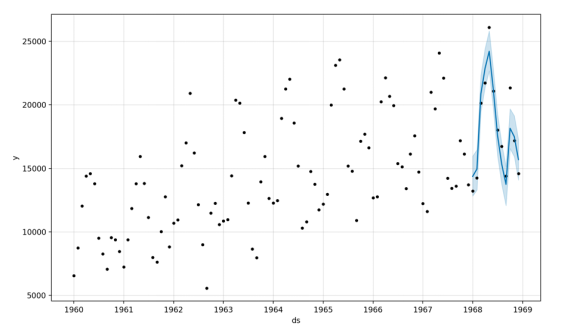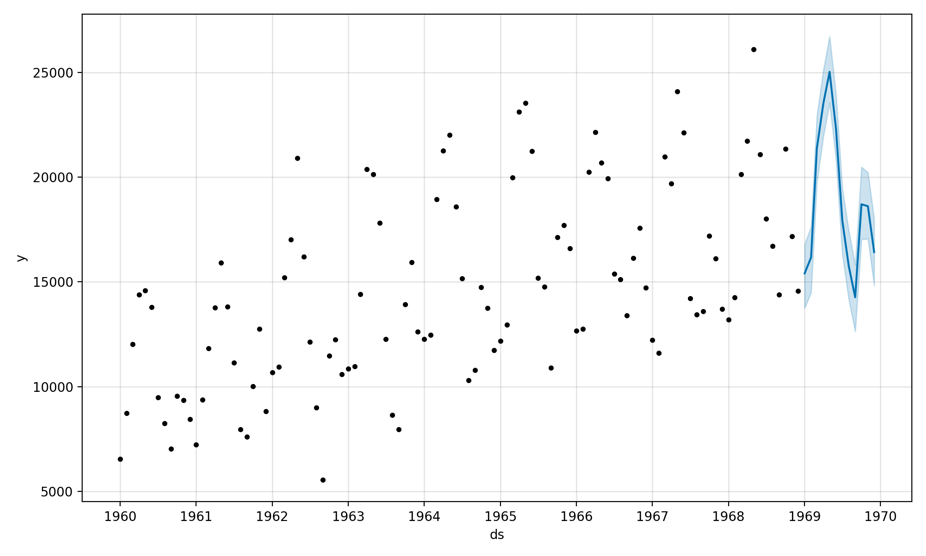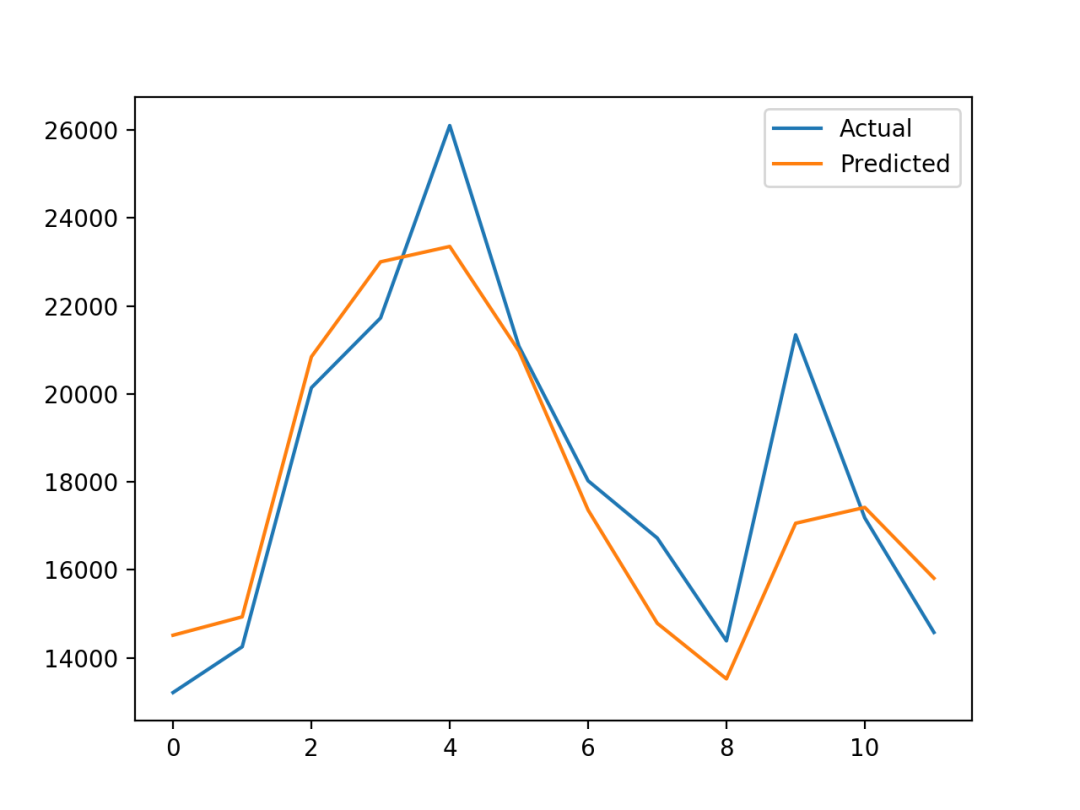# 獨家 | 手把手教你用Python的Prophet庫進行時間序列預測Prophet是一個專門為預測單變數時間序列資料集而設計的開源庫。如果你想要自動化地尋找一組好的模型超引數，從而對擁有趨勢及季節性週期變化結構的資料做出有效預測，使用Prophet來處理是一件輕而易舉的事情——它本來就是為此而設計的。

• 如何擬合Prophet模型，並使用模型進行樣本內及樣本外預測；

• 如何使用通過留出法所劃分出的不參與訓練的資料集來評估Prophet模型的效能。

• Prophet預測庫介紹

• 汽車銷量資料集

1. 載入資料並進行統計描述

2. 載入資料並進行圖表繪製

• 使用Prophet進行汽車銷量預測

1. 擬合Prophet模型

2. 進行樣本內預測

3. 進行樣本外預測

4. 手動對預測模型進行效能評估

Prophet預測庫介紹

Prophet實現的是一個可加的時間序列預測模型，支援趨勢、季節性週期變化及節假日效應。

“該模型所實現的是一個基於可加模型的時間序列資料預測過程，擬合了年度、周度、日度的季節性週期變化及節假日效應的非線性趨勢。”

— Package ‘prophet’, 2019.

Prophet的設計初衷就是簡單易用、完全自動，因此適合在公司內部場景中使用，例如預測銷量、產能等。

Prophet: forecasting at scale, 2017

https://research.fb.com/blog/2017/02/prophet-forecasting-at-scale/

``````sudo pip install fbprophet
``````

``````# check prophet version
import fbprophet
# print version number
print('Prophet %s' % fbprophet.__version__)
``````

``````Prophet 0.5
``````

Monthly Car Sales Dataset (csv)

https://raw.githubusercontent.com/jbrownlee/Datasets/master/monthly-car-sales.csv

Monthly Car Sales Dataset Description

https://raw.githubusercontent.com/jbrownlee/Datasets/master/monthly-car-sales.names

1. 載入資料並進行統計描述

Prophet要求輸入的資料為Pandas DataFrames的形式。所以我們要用Pandas庫進行資料載入和統計描述。

``````# load the car sales dataset
path = 'https://raw.githubusercontent.com/jbrownlee/Datasets/master/monthly-car-sales.csv'
# summarize shape
print(df.shape)
# show first few rows
``````

``````(108, 2)
Month  Sales
0  1960-01   6550
1  1960-02   8728
2  1960-03  12026
3  1960-04  14395
4  1960-05  14587
``````

2. 載入資料並繪製圖表

``````# load and plot the car sales dataset
from matplotlib import pyplot
path = 'https://raw.githubusercontent.com/jbrownlee/Datasets/master/monthly-car-sales.csv'
# plot the time series
df.plot()
pyplot.show()
``````1. 擬合Prophet模型

Prophet()物件會使用所傳入的引數來配置你想要的模型，例如增長和季節性週期等變化的型別。預設情況下，模型幾乎會自動找出所有的內容。

fit()函式接受時間序列資料以DataFrame的形式被傳入，同時對這個DataFrame也有特殊的格式要求：第一列必須被命名為“ds”幷包含日期資訊；第二列必須被命名為“y”幷包含觀測結果。

``````...
# prepare expected column names
df.columns = ['ds', 'y']
df['ds']= to_datetime(df['ds'])
``````

``````# fit prophet model on the car sales dataset
from pandas import to_datetime
from fbprophet import Prophet
path = 'https://raw.githubusercontent.com/jbrownlee/Datasets/master/monthly-car-sales.csv'
# prepare expected column names
df.columns = ['ds', 'y']
df['ds']= to_datetime(df['ds'])
# define the model
model = Prophet()
# fit the model
model.fit(df)
``````

``````INFO:fbprophet:Disabling weekly seasonality. Run prophet with weekly_seasonality=True to override this.
INFO:fbprophet:Disabling daily seasonality. Run prophet with daily_seasonality=True to override this.
Initial log joint probability = -4.39613
Iter      log prob        ||dx||      ||grad||       alpha      alpha0  # evals  Notes
99       270.121    0.00413718       75.7289           1           1      120
Iter      log prob        ||dx||      ||grad||       alpha      alpha0  # evals  Notes
179       270.265    0.00019681       84.1622   2.169e-06       0.001      273  LS failed, Hessian reset
199       270.283   1.38947e-05       87.8642      0.3402           1      299
Iter      log prob        ||dx||      ||grad||       alpha      alpha0  # evals  Notes
240       270.296    1.6343e-05       89.9117   1.953e-07       0.001      381  LS failed, Hessian reset
299         270.3   4.73573e-08       74.9719      0.3914           1      455
Iter      log prob        ||dx||      ||grad||       alpha      alpha0  # evals  Notes
300         270.3   8.25604e-09       74.4478      0.3522      0.3522      456
Optimization terminated normally:
Convergence detected: absolute parameter change was below tolerance
``````

2. 進行樣本內預測

``````...
# define the period for which we want a prediction
future = list()
for i in range(1, 13):
date = '1968-%02d' % i
future.append([date])
future = DataFrame(future)
future.columns = ['ds']
future['ds']= to_datetime(future['ds'])
``````

Predict()函式的計算結果是一個包含多個列的DataFrame，其中最重要的列或許是被預測的日期時間（“ds”列）、預測值（“yhat”列）以及預測值的上下限（“yhat_lower”列和“yhat_upper”列）——為預測的不確定性提供區間估計。

``````...
# summarize the forecast
``````

Prophet同樣提供了一個內建工具，用於對訓練資料預測結果進行視覺化。

``````...
# plot forecast
model.plot(forecast)
pyplot.show()
``````

``````# make an in-sample forecast
from pandas import to_datetime
from pandas import DataFrame
from fbprophet import Prophet
from matplotlib import pyplot
path = 'https://raw.githubusercontent.com/jbrownlee/Datasets/master/monthly-car-sales.csv'
# prepare expected column names
df.columns = ['ds', 'y']
df['ds']= to_datetime(df['ds'])
# define the model
model = Prophet()
# fit the model
model.fit(df)
# define the period for which we want a prediction
future = list()
for i in range(1, 13):
date = '1968-%02d' % i
future.append([date])
future = DataFrame(future)
future.columns = ['ds']
future['ds']= to_datetime(future['ds'])
# use the model to make a forecast
forecast = model.predict(future)
# summarize the forecast
# plot forecast
model.plot(forecast)
pyplot.show()
``````

``````          ds          yhat    yhat_lower    yhat_upper
0 1968-01-01  14364.866157  12816.266184  15956.555409
1 1968-02-01  14940.687225  13299.473640  16463.811658
2 1968-03-01  20858.282598  19439.403787  22345.747821
3 1968-04-01  22893.610396  21417.399440  24454.642588
4 1968-05-01  24212.079727  22667.146433  25816.191457
``````3. 進行樣本外預測

``````...
# define the period for which we want a prediction
future = list()
for i in range(1, 13):
date = '1969-%02d' % i
future.append([date])
future = DataFrame(future)
future.columns = ['ds']
future['ds']= to_datetime(future['ds'])
``````

``````# make an out-of-sample forecast
from pandas import to_datetime
from pandas import DataFrame
from fbprophet import Prophet
from matplotlib import pyplot
path = 'https://raw.githubusercontent.com/jbrownlee/Datasets/master/monthly-car-sales.csv'
# prepare expected column names
df.columns = ['ds', 'y']
df['ds']= to_datetime(df['ds'])
# define the model
model = Prophet()
# fit the model
model.fit(df)
# define the period for which we want a prediction
future = list()
for i in range(1, 13):
date = '1969-%02d' % i
future.append([date])
future = DataFrame(future)
future.columns = ['ds']
future['ds']= to_datetime(future['ds'])
# use the model to make a forecast
forecast = model.predict(future)
# summarize the forecast
# plot forecast
model.plot(forecast)
pyplot.show()
``````

``````          ds          yhat    yhat_lower    yhat_upper
0 1969-01-01  15406.401318  13751.534121  16789.969780
1 1969-02-01  16165.737458  14486.887740  17634.953132
2 1969-03-01  21384.120631  19738.950363  22926.857539
3 1969-04-01  23512.464086  21939.204670  25105.341478
4 1969-05-01  25026.039276  23544.081762  26718.820580
``````4. 手動對預測模型進行效能評估

``````...
# create test dataset, remove last 12 months
train = df.drop(df.index[-12:])
print(train.tail())
``````

``````...
# calculate MAE between expected and predicted values for december
y_true = df['y'][-12:].values
y_pred = forecast['yhat'].values
mae = mean_absolute_error(y_true, y_pred)
print('MAE: %.3f' % mae)
``````

``````...
# plot expected vs actual
pyplot.plot(y_true, label='Actual')
pyplot.plot(y_pred, label='Predicted')
pyplot.legend()
pyplot.show()
``````

``````# evaluate prophet time series forecasting model on hold out dataset
from pandas import to_datetime
from pandas import DataFrame
from fbprophet import Prophet
from sklearn.metrics import mean_absolute_error
from matplotlib import pyplot
path = 'https://raw.githubusercontent.com/jbrownlee/Datasets/master/monthly-car-sales.csv'
# prepare expected column names
df.columns = ['ds', 'y']
df['ds']= to_datetime(df['ds'])
# create test dataset, remove last 12 months
train = df.drop(df.index[-12:])
print(train.tail())
# define the model
model = Prophet()
# fit the model
model.fit(train)
# define the period for which we want a prediction
future = list()
for i in range(1, 13):
date = '1968-%02d' % i
future.append([date])
future = DataFrame(future)
future.columns = ['ds']
future['ds'] = to_datetime(future['ds'])
# use the model to make a forecast
forecast = model.predict(future)
# calculate MAE between expected and predicted values for december
y_true = df['y'][-12:].values
y_pred = forecast['yhat'].values
mae = mean_absolute_error(y_true, y_pred)
print('MAE: %.3f' % mae)
# plot expected vs actual
pyplot.plot(y_true, label='Actual')
pyplot.plot(y_pred, label='Predicted')
pyplot.legend()
pyplot.show()
``````

``````           ds      y
91 1967-08-01  13434
92 1967-09-01  13598
93 1967-10-01  17187
94 1967-11-01  16119
95 1967-12-01  13713
``````

``````MAE: 1336.814
``````Prophet庫同樣提供了一些能夠評估模型效能及繪製預測結果的自動化工具，儘管它們在本例的資料上並不是很有效。

Prophet Homepage

Prophet GitHub Project

Prophet API Documentation

Prophet: forecasting at scale, 2017

https://research.fb.com/blog/2017/02/prophet-forecasting-at-scale/

Forecasting at scale, 2017

https://peerj.com/preprints/3190/

Car Sales Dataset

https://raw.githubusercontent.com/jbrownlee/Datasets/master/monthly-car-sales.csv

Package ‘prophet’, R Documentation

https://cran.r-project.org/web/packages/prophet/prophet.pdf

• 如何擬合Prophet模型，並使用模型進行樣本內及樣本外預測；

• 如何使用通過留出法所劃分出的不參與訓練的資料集來評估Prophet模型的效能。

Time Series Forecasting With Prophet in Python

https://machinelearningmastery.com/time-series-forecasting-with-prophet-in-python/